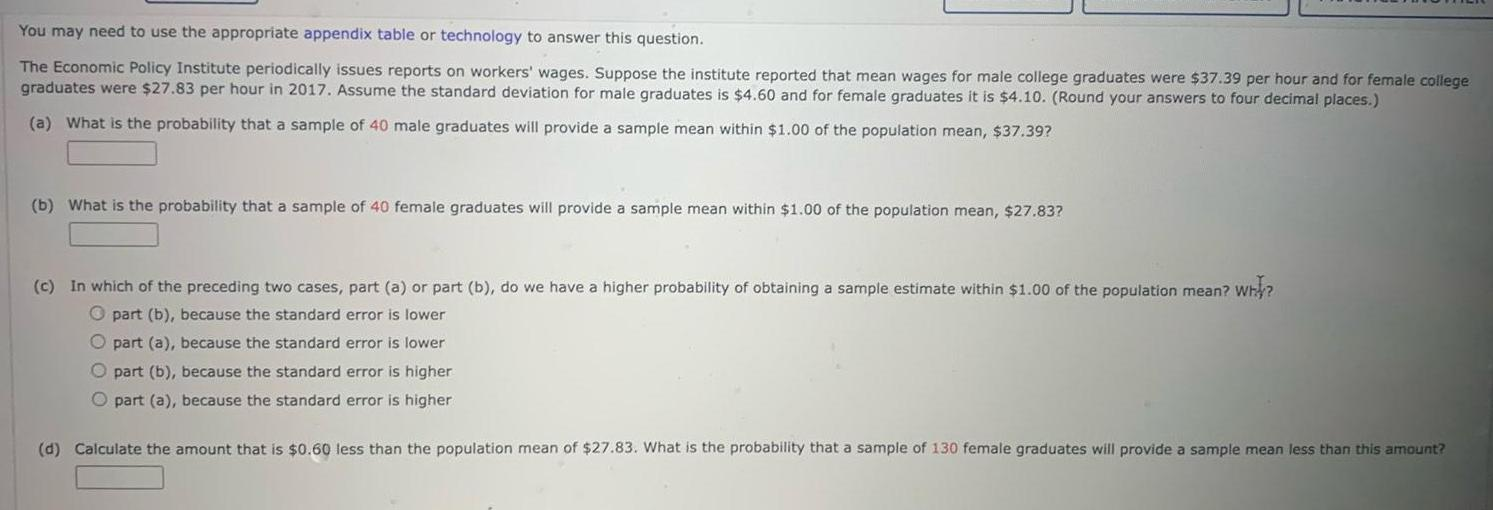Question:

# You may need to use the appropriate appendix table or

Last updated: 11/21/2023You may need to use the appropriate appendix table or technology to answer this question The Economic Policy Institute periodically issues reports on workers wages Suppose the institute reported that mean wages for male college graduates were 37 39 per hour and for female college graduates were 27 83 per hour in 2017 Assume the standard deviation for male graduates is 4 60 and for female graduates it is 4 10 Round your answers to four decimal places a What is the probability that a sample of 40 male graduates will provide a sample mean within 1 00 of the population mean 37 39 b What is the probability that a sample of 40 female graduates will provide a sample mean within 1 00 of the population mean 27 83 c In which of the preceding two cases part a or part b do we have a higher probability of obtaining a sample estimate within 1 00 of the population mean why O part b because the standard error is lower O part a because the standard error is lower O part b because the standard error is higher O part a because the standard error is higher d Calculate the amount that is 0 60 less than the population mean of 27 83 What is the probability that a sample of 130 female graduates will provide a sample mean less than this amount